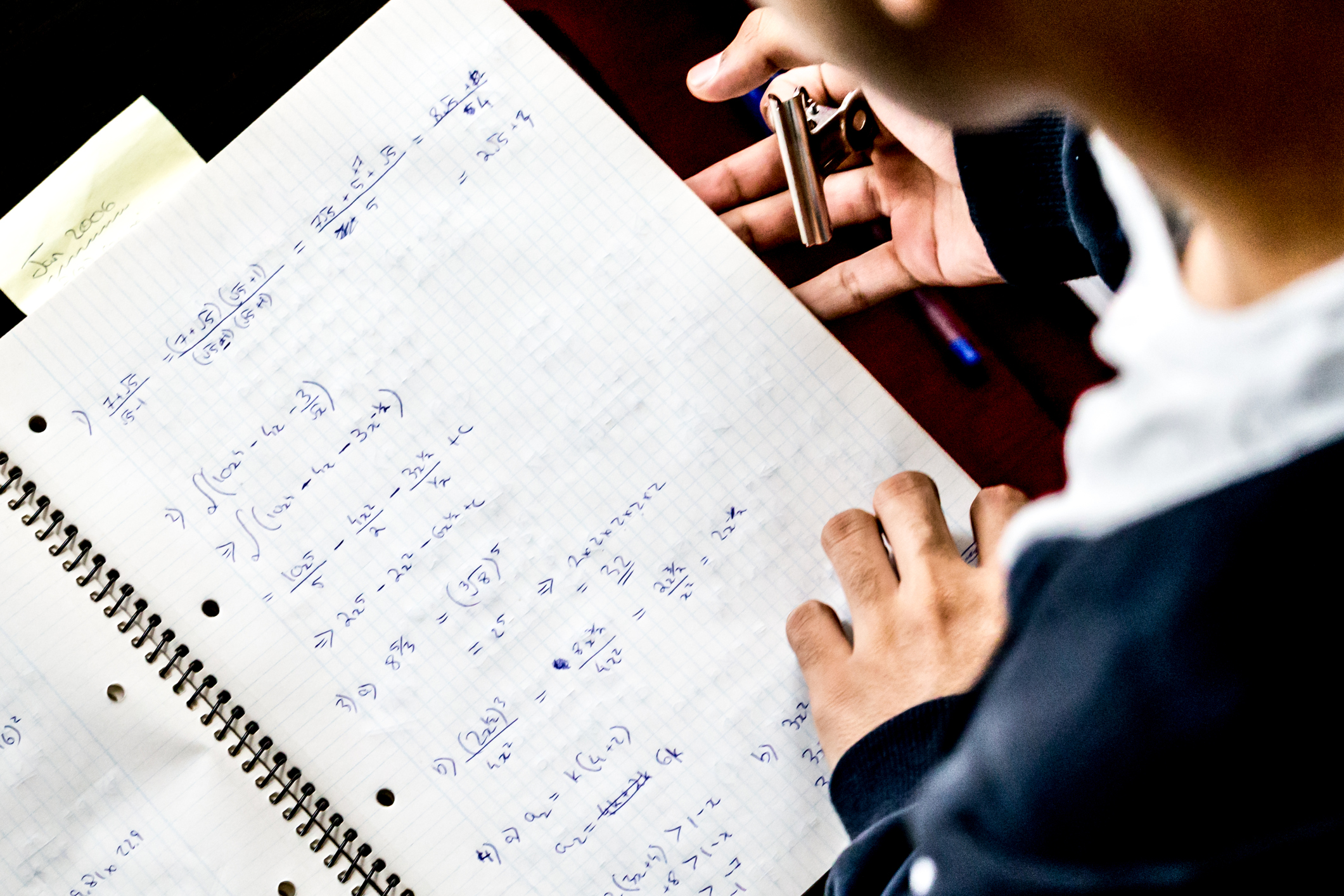Call us today: 021 156 9281

### Calculus Tutoring

One-on-one Calculus Tuition in Christchurch

#### Why Calculus Tuition with AHeadStart?

• Personalised Calculus tutoring in Christchurch - one-on-one or in small groups
• Calculus tuition for all levels - secondary (NCEA, Cambridge & IB) and adult students
• Expert Calculus tutors - high achieving, excellent communicators with a passion for learning
• Choice of Calculus tuition plans - select your desired level of guidance, including progress reports
• Convenient Christchurch locations - private home tutoring or at a convenient local library

A branch of mathematics that can be used in science, engineering
and economics to solve complicated problems.

#### Calculus

Calculus is the mathematical study of continuous change, and has historically been called "the calculus of infinitesimals", or "infinitesimal calculus". Calculus has two major subsets: differentiation (which analyses slopes of curves and related rates of change), and integration (which focuses on the aggregation of quantities, as well as the areas under curves). The fundamental theorem of calculus links differentation to integration by showing how the two processes are the opposite of each other.

It is  generally considered that Isaac Newton and Gottfried Wilhelm Leibniz developed modern Calculus during the 17th century. Today Calculus is used extensively in engineering, science, and economics.

Calculus is a stepping stone to more advanced pure and applied studies in Mathematics. The study of Calculus is designed for students intending to complete any algebraic or calculus based study at university level, such as pure mathematics, physical sciences, engineering and economics.#### NCEA Calculus

Level 3
Calculus at Level 3 involves a extension of the concepts of differentiation and integration covered in Level 2. The application of these methods is usually to describe rates of change, as well as calculating areas and volumes of curved shapes. Algebra is also extended to include imaginary numbers , which are derived from taking the square root of -1. The topic of trigonometry becomes more applied, with sin and cos functions used to describe circular motion. Some students will also learn about conic functions -  circles, ellipses, hyperbolae and parabola, which should have been covered in Level 2.

“Reinforced basic concepts and showed how to answer exam questions. He got the Calculus prize at end of year prize giving!”

Alex’s mother
Year 13, Level 3 Calculus

"Melanie fitted my daughter in an already packed schedule of hers. She went the extra mile to make sure she understood every concept in calculus. The result of her effective approach was a very confident student. My daughter just went from strength to strength, passed her internal exams, feels confident about success in the end of year exams and is absolutely motivated. Thank you, Melanie!"

Nastassja’s mother Henriette
Year 13, Level 3 Calculus

### CALCULUS Resources

All of the following book resources can be purchased at leading bookshops or the following websites:

ESA Publications Store: https://esa.co.nz/

StudyPass: https://studypass.com/

Pearson: http://www.pearsoned.co.nz

Level 3
ESA Level 3 Calculus Study Guide
ESA Level 3 Calculus Learning Workbook
ESA Level 3 Calculus AME Workbook
ESA Level 3 Scholarship Calculus AME Workbook

StudyPass NCEA Level 3 Calculus

Pearson Delta Mathematics: NCEA Level 3
Pearson Delta Mathematics Workbook: NCEA Level 3